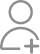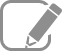Take Class 9 Tuition from the Best Tutors

•Affordable fees
•1-1 or Group class
•Flexible Timings
•Verified Tutors

Search in

# Write the distribution of electrons in carbon and sodium atoms.

Learn Intext Exercise 4.4Follow 2AnswerThe total number of electrons in a carbon atom is 6. The distribution of electrons in carbon atom is given by: First orbit or K-shell = 2 electrons Second orbit or L-shell = 4 electrons Or, we can write the distribution of electrons in a carbon atom as 2, 4. The total number of electrons in a sodium...

The total number of electrons in a carbon atom is 6. The distribution of electrons in carbon atom is given by:

First orbit or K-shell = 2 electrons

Second orbit or L-shell = 4 electrons

Or, we can write the distribution of electrons in a carbon atom as 2, 4.

The total number of electrons in a sodium atom is 11. The distribution of electrons in sodium atom is given by:

First orbit or K-shell = 2 electrons

Second orbit or L-shell = 8 electrons

Third orbit or M-shell = 1 electron

Or, we can write distribution of electrons in a sodium atom as 2, 8, 1.

The total number of electrons in a carbon atom is 6. The distribution of electrons in carbon atom is given by: First orbit or K-shell = 2 electrons Second orbit or L-shell = 4 electrons Or, we can write the distribution of electrons in a carbon atom as 2, 4. The total number of electrons in a sodium...

The total number of electrons in a carbon atom is 6. The distribution of electrons in carbon atom is given by:

First orbit or K-shell = 2 electrons

Second orbit or L-shell = 4 electrons

Or, we can write the distribution of electrons in a carbon atom as 2, 4.

The total number of electrons in a sodium atom is 11. The distribution of electrons in sodium atom is given by:

First orbit or K-shell = 2 electrons

Second orbit or L-shell = 8 electrons

Third orbit or M-shell = 1 electron

Or, we can write distribution of electrons in a sodium atom as 2, 8, 1.

Now ask question in any of the 1000+ Categories, and get Answers from Tutors and Trainers on UrbanPro.com

Find Class 9 Tuition near you

Looking for Class 9 Tuition ?

Learn from the Best Tutors on UrbanPro

Are you a Tutor or Training Institute?

Join UrbanPro Today to find students near you
X

### Looking for Class 9 Tuition Classes?

The best tutors for Class 9 Tuition Classes are on UrbanPro

• Select the best Tutor
• Book & Attend a Free Demo
• Pay and start Learning### Take Class 9 Tuition with the Best Tutors

The best Tutors for Class 9 Tuition Classes are on UrbanPro